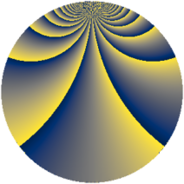# Properties

 Label 684.3.cgLevel $684$ Weight $3$ Character orbit 684.cg Rep. character $\chi_{684}(55,\cdot)$ Character field $\Q(\zeta_{18})$ Dimension $588$ Sturm bound $360$

# Related objects

## Defining parameters

 Level: $$N$$ $$=$$ $$684 = 2^{2} \cdot 3^{2} \cdot 19$$ Weight: $$k$$ $$=$$ $$3$$ Character orbit: $$[\chi]$$ $$=$$ 684.cg (of order $$18$$ and degree $$6$$) Character conductor: $$\operatorname{cond}(\chi)$$ $$=$$ $$76$$ Character field: $$\Q(\zeta_{18})$$ Sturm bound: $$360$$

## Dimensions

The following table gives the dimensions of various subspaces of $$M_{3}(684, [\chi])$$.

Total New Old
Modular forms 1488 612 876
Cusp forms 1392 588 804
Eisenstein series 96 24 72

## Trace form

 $$588q + 6q^{2} - 12q^{4} + 12q^{5} + 3q^{8} + O(q^{10})$$ $$588q + 6q^{2} - 12q^{4} + 12q^{5} + 3q^{8} + 51q^{10} + 12q^{13} - 27q^{14} + 36q^{16} + 12q^{17} + 42q^{20} - 18q^{22} - 12q^{25} - 69q^{26} - 216q^{28} + 12q^{29} - 249q^{32} - 108q^{34} - 24q^{37} + 252q^{38} + 330q^{40} + 216q^{41} + 363q^{44} + 132q^{46} + 1800q^{49} + 120q^{50} + 9q^{52} - 12q^{53} + 306q^{56} + 60q^{58} + 396q^{61} + 468q^{62} + 75q^{64} - 114q^{65} + 240q^{68} - 765q^{70} - 444q^{73} - 363q^{74} + 570q^{76} + 756q^{77} + 69q^{80} + 183q^{82} - 924q^{85} - 768q^{86} - 75q^{88} + 372q^{89} - 1092q^{92} + 1074q^{94} + 96q^{97} + 603q^{98} + O(q^{100})$$

## Decomposition of $$S_{3}^{\mathrm{new}}(684, [\chi])$$ into newform subspaces

The newforms in this space have not yet been added to the LMFDB.

## Decomposition of $$S_{3}^{\mathrm{old}}(684, [\chi])$$ into lower level spaces

$$S_{3}^{\mathrm{old}}(684, [\chi]) \cong$$ $$S_{3}^{\mathrm{new}}(76, [\chi])$$$$^{\oplus 3}$$$$\oplus$$$$S_{3}^{\mathrm{new}}(228, [\chi])$$$$^{\oplus 2}$$